# How to set the Background Color of the RichTextBox in C#?

In C#, RichTextBox control is a textbox which gives you rich text editing controls and advanced formatting features also includes a loading rich text format (RTF) files. Or in other words, RichTextBox controls allows you to display or edit flow content, including paragraphs, images, tables, etc. In RichTextBox, you are allowed to change the background color of the RichTextBox control using BackColor Property which makes your RichTextBox control more attractive. You can set this property in two different ways:

1. Design-Time: It is the easiest way to set the background color of the RichTextBox as shown in the following steps:

• Step 1: Create a windows form as shown in the below image:
Visual Studio -> File -> New -> Project -> WindowsFormApp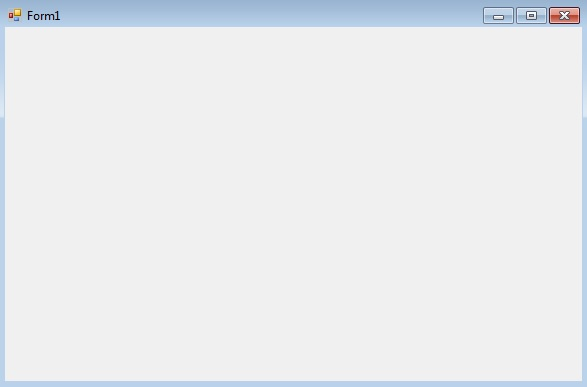• Step 2: Drag the RichTextBox control from the ToolBox and drop it on the windows form. You are allowed to place a RichTextBox control anywhere on the windows form according to your need.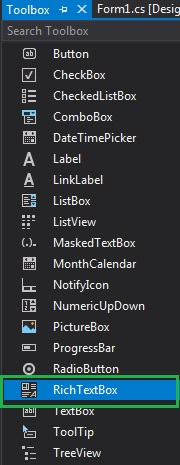• Step 3: After drag and drop you will go to the properties of the RichTextBox control set the background color of the RichTextBox control.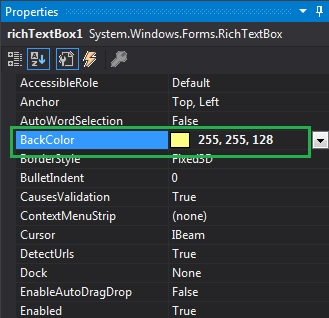Output: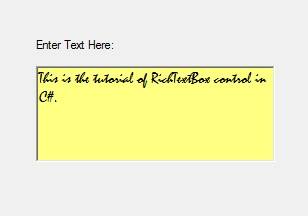2. Run-Time: It is a little bit trickier than the above method. In this method, you can sort the elements present in the RichTextBox control programmatically with the help of given syntax:

`public override System.Drawing.Color BackColor { get; set; }`

Here, Color indicates the background color of the RichTextBox. The following steps show how to set the BackColor property of the RichTextBox dynamically:

• Step 1: Create a RichTextBox using the RichTextBox() constructor is provided by the RichTextBox class.
```// Creating RichTextBox using RichTextBox class constructor
RichTextBox rbox = new RichTextBox();
```
• Step 2: After creating RichTextBox, set the BackColor Property of the RichTextBox provided by the RichTextBox class.
```// Setting the background color
rbox.BackColor = Color.Aqua;
```
• Step 3: And last add this RichTextBox control to the form using Add() method.
```// Add this RichTextBox to the form
```

Example:

 `using` `System; ` `using` `System.Collections.Generic; ` `using` `System.ComponentModel; ` `using` `System.Data; ` `using` `System.Drawing; ` `using` `System.Linq; ` `using` `System.Text; ` `using` `System.Threading.Tasks; ` `using` `System.Windows.Forms; ` ` `  `namespace` `WindowsFormsApp30 { ` ` `  `public` `partial` `class` `Form1 : Form { ` ` `  `    ``public` `Form1() ` `    ``{ ` `        ``InitializeComponent(); ` `    ``} ` ` `  `    ``private` `void` `Form1_Load(``object` `sender, EventArgs e) ` `    ``{ ` `        ``// Creating and setting the  ` `        ``// properties of the label ` `        ``Label lb = ``new` `Label(); ` `        ``lb.Location = ``new` `Point(251, 70); ` `        ``lb.Text = ``"Enter Text"``; ` ` `  `        ``// Adding this label in the form ` `        ``this``.Controls.Add(lb); ` ` `  `        ``// Creating and setting the  ` `        ``// properties of the RichTextBox ` `        ``RichTextBox rbox = ``new` `RichTextBox(); ` `        ``rbox.Location = ``new` `Point(236, 97); ` `        ``rbox.BackColor = Color.Aqua; ` `        ``rbox.Text = ``"!..Welcome to GeeksforGeeks..!"``; ` ` `  `        ``// Adding this RichTextBox in the form ` `        ``this``.Controls.Add(rbox); ` `    ``} ` `} ` `} `

Output: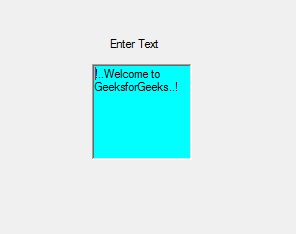My Personal Notes arrow_drop_up

## Recommended Posts:

Article Tags :

Be the First to upvote.

Please write to us at contribute@geeksforgeeks.org to report any issue with the above content.